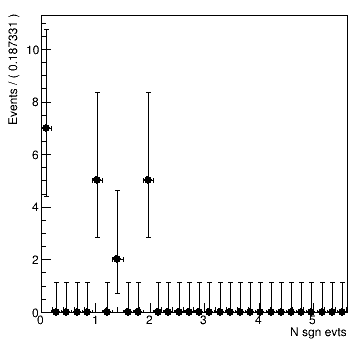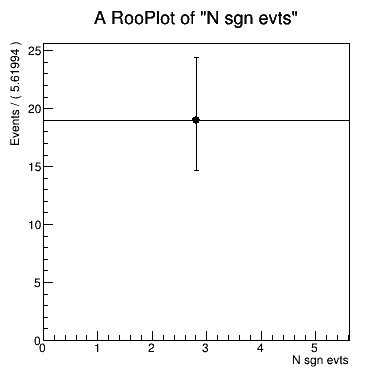# Process fit results when using RooMCStudy

Hello experts!

I am now discovering for myself a `RooMCStudy` class to perform a LEE study.

Up to this point I was able to successfully generate a set of ToyMC samples, fit them and overview simple results (please see my macro).
toyMC.C (4.7 KB)

Although, I would like to extract some additional information from the set of fits, and this I am failing to succeed in. What I want to do is:

1. Catch a particular ToyMC data sample and plot it’s fit (including separate fit components):
I retrieve a randomly-selected ToyMC sample:

RooAbsData *gendata_i = mcstudy->genData(iMCtestSample);

I then retrieve corresponding `RooFitResult`:

const RooFitResult *fitres_i = mcstudy->fitResult(iMCtestSample);

Now I want to forward final values of floating parameters to a PDF and plot it over the sample. I also want to see all separate PDF components plotted.

2. Access the values of fit parameters to work with them.
I want now to get the nevt values from the fits to count the number of cases, when it acceded a particular threshold value. But I could not find a way to get the values from the fits to iterate over them. The only thing I could find within the attributes of `RooFitResult` is

fitres_i->floatParsFinal().at(i)->Print()

In this way, I can at least see the desired parameters. But I could not find for any analog of `getVal()` for `RooAbsArg` (which is a data type for `fitres_i->floatParsFinal().at(i)`). I also was not able to find a way to access the value of a particular fit parameter by referring to it by its name, I mean something like `.at("nsig")`.

This is a short overview of the problems I am facing. Please let me know if any additional information/clarification required.

I will be grateful for any help with any of these questions.

Dmytro.

Hi @mc.dmytro, sorry for the late reply!

I have written a little standalone demonstration code to show you how you can use the RooFitResult to assign the values of a given fit result to the PDF parameters, and also how to get the values from the fit result. I think the functions I explain here help you to do exactly what you want with the RooMCStudy!

Cheers,
Jonas

``````// Observables and parameters
RooRealVar x("x", "x", -10, 10);
RooRealVar mean("mean", "mean of gaussian", 1, -10, 10);
RooRealVar sigma("sigma", "width of gaussian", 1, 0.1, 10);

// PDF
RooGaussian gauss("gauss", "gaussian PDF", x, mean, sigma);

// Toy dataset
std::unique_ptr<RooDataSet> data{gauss.generate(x, 1000)};

// Keeping a snaphot of the original parameter values and errors
RooArgSet params;
gauss.getParameters(data->get()); // need to tell getParameter" what the observables are
RooArgSet originalParams;
params.snapshot(originalParams);

// Fit pdf to data
std::unique_ptr<RooFitResult> result{gauss.fitTo(*data, RooFit::Save(), RooFit::PrintLevel(-1))};
result->Print();

// After the fit, the parameters will have post-fit values and errors
mean.Print();
sigma.Print();

// We can reset the values and errors when assigning between RooArgSets
params = originalParams;
mean.Print();
sigma.Print();

// Let's assign again the post-fit parameters, obtained from the fit result
// object. Here, you could also use the fit result returned by
// RooMCStudy::fitResult(i). You can then plot the PDF together with
// the corresponding toy dataset.
params = result->floatParsFinal();
mean.Print();
sigma.Print();

// To access the values from the fit result, you need to cast the elements in
// floatParsFinal() to RooRealVar first. To get the elements from the
// RooArgSet, you can use the bracket operator. Don't forget to use reference
// types (the "&") here to about copying.
RooArgSet const& parsFinal = result->floatParsFinal();
auto& meanFinal = static_cast<RooRealVar&>(parsFinal["mean"]);
auto& sigmaFinal = static_cast<RooRealVar&>(parsFinal["sigma"]);

// Inspect the variable values and errors:
std::cout << meanFinal.getVal() << "   " << meanFinal.getError() << std::endl;
std::cout << sigmaFinal.getVal() << "   " << sigmaFinal.getError() << std::endl;
``````

Dear @jonas,

thank you very much for your reply. I was able to implement a couple of features I’ve been struggling with before. Although, I would still like to clarify one thing additionally and to raise a newly-arose question.

1. Exporting `RooFitResults` to a fit function in order to plot it.
In one of the comments lines, you said: “You can then plot the PDF together with the corresponding toy dataset.”
Could you please explain how to do it in a right way?
In the previous answer, you showed a way to export parameters to `RooArgSet` and simple operations with the created `RooArgSet`. But, if I understand correctly, in order to plot a PDF, one needs to assign values extracted from `RooFitResults` to the floating parameters. Although I was able to do it by writing the lines given below, I feel that there is a better, shorter, way to do it.
``````// Let's pick up a random test sample
RooAbsData *gendata_k = mcstudy->genData(iMCtestSample);
const RooFitResult *fitres_k = mcstudy->fitResult(iMCtestSample); // get fit result of a random toyMC
RooArgSet const& pars_k = fitres_k->floatParsFinal();

// Record fit values for the respective fit
auto& mean_k  = static_cast<RooRealVar&>(pars_k["mean_fit"]);
auto& sigma_k = static_cast<RooRealVar&>(pars_k["sigma_fit"]);
auto& a0_k    = static_cast<RooRealVar&>(pars_k["a0_fit"]);
auto& a1_k    = static_cast<RooRealVar&>(pars_k["a1_fit"]);
auto& a2_k    = static_cast<RooRealVar&>(pars_k["a2_fit"]);
auto& a3_k    = static_cast<RooRealVar&>(pars_k["a3_fit"]);
auto& a4_k    = static_cast<RooRealVar&>(pars_k["a4_fit"]);
auto& a5_k    = static_cast<RooRealVar&>(pars_k["a5_fit"]);
auto& nsig_k  = static_cast<RooRealVar&>(pars_k["nsig"]);
auto& nbkg_k  = static_cast<RooRealVar&>(pars_k["nbkg"]);

//And set floating parameters according to these values
mean_fit.setVal(mean_k.getVal());
sigma_fit.setVal(sigma_k.getVal());
a0_fit.setVal(a0_k.getVal());
a1_fit.setVal(a1_k.getVal());
a2_fit.setVal(a2_k.getVal());
a3_fit.setVal(a3_k.getVal());
a4_fit.setVal(a4_k.getVal());
a5_fit.setVal(a5_k.getVal());
nsig.setVal(nsig_k.getVal());
nbkg.setVal(nbkg_k.getVal());
fit.plotOn(frame4);
fit.plotOn(frame4,Components(RooArgSet(bw_fit)),LineColor(kYellow-2),LineStyle(kDashed));
fit.plotOn(frame4,Components(RooArgSet(cpol_fit)),LineColor(kRed),LineStyle(kDashed));
``````
1. Missing statistics when plotting `RooMCStudy` results.
I mentioned that if, for example, I generate 50 toyMC samples and then plot the distribution of fit parameters, e.g. nsig, I get the total number of entries in a histogram, which is less than 50 (19 in my particular example). It can be mentioned by:
• counting entries (see first plot)
• pushing all values in a single bin (see second plot)
• or simply by printing all 50 nsig values in which high values in [3,5] interval exist but are missing in the plot.

What might be a reason for that?toyMC.C (7.9 KB)

1. Actually with the `params = result->floatParsFinal();` line in my script, I was already reassigning all the parameter values. When you assign one RooArgSet to another, you sync the values of the left hand side with the right hand side. So in your code, all you need to do is:
``````// Let's pick up a random test sample
RooAbsData *gendata_k = mcstudy->genData(iMCtestSample);
const RooFitResult *fitres_k = mcstudy->fitResult(iMCtestSample); // get fit result of a random toyMC

// The parameters in the PDF
RooArgSet observables{mass}; // "mass" in your case
RooArgSet pars;
fit.getParameters(&observables, pars);

// Set parameter values to values for a specific fit
pars = fitres_k->floatParsFinal();;

fit.plotOn(frame4);
fit.plotOn(frame4,Components(RooArgSet(bw_fit)),LineColor(kYellow-2),LineStyle(kDashed));
fit.plotOn(frame4,Components(RooArgSet(cpol_fit)),LineColor(kRed),LineStyle(kDashed));
``````
1. There are so few entries because if a fit doesn’t converge, the result will not be saved. Apparently of your 50 toy datasets, only 19 are converging. The reason for the bad convergence is how you are defining the Chebychev background PDF. You are adding together these polynomials here: Chebyshev polynomials - Wikipedia. According to the RooChebychev docs, the first coefficient is fixed to zero, and you give the coefficients for T1 onward.

Now, each of these base polynomials has values between -1 and 1, and you add many of them with coefficients between -1 and 1. The phase space where the full polynomial is positive is very small, so the minimizer often ends up in a phase space with negative PDF values, which are undefined and can cause the fit to fail. To fix this, you can maybe add another constant background to your model, such that the full PDF can not easily become negative.

I hope that helps you to continue for now!

Jonas

Dear @jonas,

thank you very much for help. The technical part did work for me, which is wonderful. The Chebyshev story is more complicated. I’ve been struggling with this negatively-defined fit for some time, even created a separate thread here:

In that thread, I’ve been wondering if it is a taboo for a fit curve to enter the negative region. And I think you just confirmed it.

Just like you suggested, one can try to pull the fit from the below 0 region by adding another component to the PDF. But up to now I could not come to an idea, which will be meaningful from the prospective of physics.

Anyway, I thank you very much for being extremely helpful.

Best regards,
Dmytro.

This topic was automatically closed 14 days after the last reply. New replies are no longer allowed.